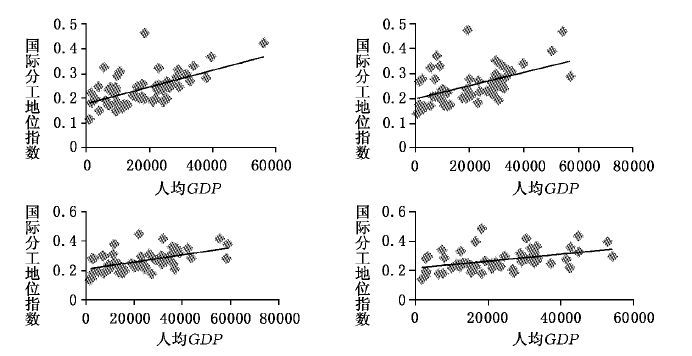﻿ 中国国际分工地位的再评估——基于出口技术复杂度与国内增加值双重视角的分析
 财经研究2016, Vol. 42Issue (6): 40-510

#### 文章信息

Su Qingyi.

Re-evaluation of China's Position in International Division from the Dual Perspectives of Export Technological Sophistication and Domestic Value Added

Journal of Finance and Economics, 2016, 42(6): 40-51.

### 文章历史

Re-evaluation of China's Position in International Division from the Dual Perspectives of Export Technological Sophistication and Domestic Value Added
Su Qingyi
Institute of World Economics and Politics, Chinese Academy of Social Sciences, Beijing 100732, China
Abstract: Reasonable evaluation of current China's position in international division is the key basis of judging China's export strengths and weaknesses as well as improving its position in international division. This paper constructs a new index of position in international division possessing both product and domestic value added attributes by integrating export technological sophistication and domestic value added.Then it constructs a theoretical model about understanding the differences in positions in international division based on input-output framework, and re-evaluates China's position in international division. It draws the results as follows:firstly, at present, China's position in international division is in a rank of the most backward economies when considering services export; Asian emerging economies' positions in international division are generally not high, and developed and resources-rich economies are in relatively higher positions in international division; secondly, from 1995 to 2009, the differences in positions in international division between China and US, India, Brazil have widened, while the difference in positions in international division between China and Germany is relatively stable, and the difference in positions in international division between China and Japan has been reduced to some extent; thirdly, the main reason for China's backward position in international division lies in export structure, namely China's ratio of services export is relatively low, and the reduction in domestic value added also has the increasingly important effect; fourthly, the evolution of the index concerning China's position in international division stems from industry attribute effect and the effect of domestic value added, and the export structure effect is very low. Therefore, the adjustment to export structure and the enhancement of domestic value added ratio are two important ways for China to improve its position in international division.
Key words: global value chain    position in international division    export structure    domestic value-added ratio

 $GVC{P_i} = {\sum _k}\frac{{{x_{ik}}}}{{{X_i}}}DVA{R_{ik}}H{L_k}$ (1)

 $GVC{P_i} = {\sum _k}\frac{{{x_{ik}}}}{{{x_i}}}DVA{R_{ik}}PROD{Y_k}$ (2)中间使用 最终使用 总产出 国家1 国家2 国家1 国家2 产品A 产品B 产品A 产品B 中间投入 国家1 产品A x11AA x11AB 0 0 y11A y12A x1A 产品B x11BA x11BB 0 0 y11B y12B x1B 国家2 产品A 0 0 x22AA x22AB y21A y22A x2A 产品B x21BA x21BB x22BA x22BB y21B y22B x2B 增加值 va1A va1B va2A va2B 总投入 x1A x1B x2A x2B

 $GVC{P_{1A}} = 1 - \left[ {\frac{{x_{21}^{BA}}}{{x_1^A}}\left( {1 + \frac{{x_{11}^{AA}}}{{x_1^A}}} \right) + \frac{{x_{21}^{BA}}}{{x_1^B}}\frac{{x_{11}^{BA}}}{{x_1^A}}} \right],DVA{R_{1A}} ＜ 1 = DVA{R_{2A}}$ (3)
 $GVC{P_{1B}} = 1 - \left[ {\frac{{x_{21}^{BA}}}{{x_1^A}}\frac{{x_{11}^{AB}}}{{x_1^B}} + \frac{{x_{21}^{BB}}}{{x_1^B}}\left( {1 + \frac{{x_{11}^{BB}}}{{x_1^B}}} \right)} \right],DVA{R_{1B}} ＜ 1 = DVA{R_{2B}}$ (4)

 $GVC{P_1} = \frac{{y_{12}^A}}{{y_{12}^A + y_{12}^B}}DVA{R_{1A}}PROD{Y_A} + \frac{{y_{12}^B}}{{y_{12}^A + y_{12}^B}}DVA{R_{1B}}PROD{Y_B}$ (5)
 $GVC{P_2} = \frac{{y_{21}^A}}{{y_{21}^A + y_{21}^B + x_{21}^{BA} + x_{21}^{BB}}}PROD{Y_A} + \frac{{y_{21}^B + x_{21}^{BA} + x_{21}^{BB}}}{{y_{21}^A + y_{21}^B + x_{21}^{BA} + x_{21}^{BB}}}PROD{Y_B}$ (6)

 $PRODY_k^{{\rm{new}}} = \frac{{PRODY{}_k - PROD{Y_{min}}}}{{PRODY{}_{max} - PROD{Y_{min}}}}$ (7)

(二) 世界主要经济体的国际分工地位的评估结果1995 2000 2005 2008 2009 沙特 0.625 0.462 0.475 0.445 0.483 英国 0.412 0.321 0.351 0.412 0.417 俄罗斯 0.444 0.306 0.370 0.376 0.395 澳大利亚 0.405 0.284 0.326 0.356 0.362 美国 0.455 0.328 0.339 0.348 0.357 中国香港 0.302 0.267 0.332 0.350 0.349 南非 0.462 0.322 0.323 0.299 0.339 加拿大 0.382 0.243 0.285 0.310 0.318 西班牙 0.362 0.256 0.270 0.309 0.314 日本 0.503 0.313 0.297 0.298 0.295 印度尼西亚 0.362 0.245 0.276 0.278 0.293 巴西 0.384 0.242 0.276 0.293 0.284 德国 0.409 0.264 0.266 0.286 0.283 印度 0.294 0.219 0.267 0.278 0.280 意大利 0.340 0.230 0.238 0.273 0.276 法国 0.377 0.252 0.264 0.268 0.268 荷兰 0.276 0.196 0.238 0.242 0.245 葡萄牙 0.280 0.208 0.236 0.235 0.243 阿根廷 0.335 0.237 0.235 0.223 0.239 墨西哥 0.342 0.213 0.233 0.245 0.234 新加坡 0.276 0.182 0.191 0.206 0.216 马来西亚 0.256 0.182 0.200 0.218 0.215 韩国 0.339 0.204 0.203 0.209 0.205 菲律宾 0.298 0.147 0.155 0.183 0.190 中国台湾 0.283 0.194 0.181 0.174 0.182 泰国 0.288 0.174 0.168 0.177 0.178 中国 0.318 0.194 0.166 0.186 0.173 越南 0.255 0.179 0.173 0.143 0.161 注:根据2009年国际分工地位指数由高到低排列。图 1 人均GDP和国际分工地位的散点图
(三) 中国与代表性国家之间国际分工地位差异的来源

 $\begin{array}{ccccc} GVC{P_{china}} - GVC{P_{other}} = & \frac{{{\sum _k}\left( {{r_{k,other}}DVA{R_{k,china}}PROD{Y_k} - {r_{k,other}}DVA{R_{k,other}}PROD{Y_k}} \right)}}{{{\rm{国内增加值校应}}\left( {DVAR{E_1}} \right)}}\\ + \frac{{{\sum _k}\left( {{r_{k,china}}DVA{R_{k,china}}PROD{Y_k} - {r_{k,other}}DVA{R_{k,china}}PROD{Y_k}} \right)}}{{{\rm{出口结构效应}}\left( {ES{E_1}} \right)}}\\ = \frac{{{\sum _k}\left( {{r_{k,china}}DVA{R_{k,china}}PROD{Y_k} - {r_{k,china}}DVA{R_{k,other}}PROD{Y_k}} \right)}}{{{\rm{国内增加值校应}}\left( {DVAR{E_2}} \right)}}\\ + \frac{{{\sum _k}\left( {{r_{k,china}}DVA{R_{k,other}}PROD{Y_k} - {r_{k,other}}DVA{R_{k,other}}PROD{Y_k}} \right)}}{{{\rm{出口结构效应}}\left( {ES{E_2}} \right)}} \end{array}$ (8)

1.中国与美国之间的差异。1995-2009年中国的国际分工地位均低于美国,且落后程度逐步加大,从1995年的0.138扩大到2009年的0.184。分解结果表明,这种差异主要来源于出口结构效应,但国内增加值效应的贡献度在不断上升。这说明中国的出口结构还需要调整和优化,需要提升高端制造业和服务业的比重。一个显而易见的例子是,美国的金融服务业远远领先于中国。同时,中国出口产业的国内增加值率与美国之间的差距在逐步拉大,这越发阻碍了中国追赶美国的国际分工地位。事实上,根据TiVA数据库的计算结果,1995年中国国内增加值率低于美国仅3.51个百分点,而2009年则扩大到了21.34个百分点。

2.中国与日本之间的差异。与中美之间分工地位差异不同的是,总体而言,1995-2009年中国与日本之间的国际分工地位差异有所缩小。1995-2000年的这种分工差异主要源于出口结构效应,即中国出口结构相比于日本而言还不够高端。但是,2005-2009年的国内增加值效应变得日益重要,与出口结构效应不相上下,甚至在2005年超过了出口结构效应的贡献度。这说明中国的出口结构在不断地追赶日本,并随着时间的推进而有所优化。但是,与美国类似,中国与日本之间的国内增加值率的差异也在不断扩大。

3.中国与德国之间的差异。1995-2009年中国与德国之间的国际分工地位差异并没有明显被拉大,甚至在2000年有所降低。2005-2009年中国与德国之间的国际分工地位差异一直比较稳定。中国与德国之间的国际分工地位差异主要来源于出口结构效应,国内增加值效应的贡献度较低。国内增加值效应甚至在1995年和2000年促成了中国向上追赶德国的国际分工地位。国内增加值率的计算结果证实了这一点,1995年和2000年的中国国内增加值率分别高于德国6.82和5.59个百分点;而在2005年以后,中国的国内增加值率均低于德国。

4.中国与印度之间的差异。1995年中国的国际分工地位略微高于印度,但2000-2009年中国的分工地位一直低于印度,而且有被印度拉大的趋势。其背后最主要的原因是出口结构效应。中国和印度的出口走出了两条不同的路径。2000年以来,印度的服务业发展迅猛,并一直依赖服务业出口,而中国的制造业更为强大。1995年中国和印度的服务业出口占自身总出口的比重分别是13.29%和18.48%,差距并不明显;但到了2009年,印度的服务业出口比重已经上升到了37.14%,而中国的服务业出口比重却下降到了9.41%。根据前面对产业技术复杂度的计算,从国际分工地位的角度来讲,发展服务业更能提升国际分工地位,这也是印度的国际分工地位高于中国的主要原因。

5.中国与巴西之间的差异。1995-2009年中国的国际分工地位持续低于巴西。相比于1995年,2000年中国与巴西之间的落后程度有所缩小;但2005年后又有所拉大,并于2008年和2009年保持稳定。1995年和2000年的这种差异主要来源于出口结构效应,但是2005-2009年的差异主要来源于国内增加值效应。一方面,巴西采掘业出口占比较高,1995年巴西和中国的采掘业出口占比分别是7.71%和1.99%,2009年巴西的采掘业出口占比上升到了11.44%,而中国的采掘业出口占比却下降到了0.60%;另一方面,随着中国制造业出口规模的持续扩大,中国的国内增加值率却较低,而巴西由于出口较多原材料而拥有较高国内增加值率,中国与巴西之间的国内增加值率差异在逐步拉大,造成中国的国际分工地位低于巴西。1995年中国的国内增加值率落后巴西仅2.17个百分点,而2009年中国的国内增加值率较巴西低了23.6个百分点。

(一) 中国自身国际分工地位的演进

(二) 各因素对中国国际分工地位变化的贡献度

 $\begin{array}{ccccc} GVC{P_{t1}} - GVC{P_{t0}} = & \frac{{{\sum _k}\left( {{r_{k,t1}}DVA{R_{k,t0}}PROD{Y_{k,t0}} - {r_{k,t0}}DVA{R_{k,t0}}PROD{Y_{k,t0}}} \right)}}{{{\rm{出口结构效应}}\left( {ES{E_1}} \right)}}\\ + \frac{{{\sum _k}\left( {{r_{k,t1}}DVA{R_{k,t1}}PROD{Y_{k,t0}} - {r_{k,t1}}DVA{R_{k,t0}}PROD{Y_{k,t0}}} \right)}}{{{\rm{国内增加值效应}}\left( {DVAR{E_1}} \right)}}\\ + \frac{{{\sum _k}\left( {{r_{k,t1}}DVA{R_{k,t1}}PROD{Y_{k,t1}} - {r_{k,t1}}DVA{R_{k,t1}}PROD{Y_{k,t0}}} \right)}}{{{\rm{产业属性效应}}\left( {P{E_1}} \right)}}\\ = \frac{{{\sum _k}\left( {{r_{k,t1}}DVA{R_{k,t1}}PROD{Y_{k,t1}} - {r_{k,t0}}DVA{R_{k,t1}}PROD{Y_{k,t1}}} \right)}}{{{\rm{出口结构效应}}\left( {ES{E_2}} \right)}}\\ + \frac{{{\sum _k}\left( {{r_{k,china}}DVA{R_{k,other}}PROD{Y_k} - {r_{k,other}}DVA{R_{k,other}}PROD{Y_k}} \right)}}{{{\rm{国内增加值效应}}\left( {DVAR{E_2}} \right)}}\\ + \frac{{{\sum _k}\left( {{r_{k,t0}}DVA{R_{k,t0}}PROD{Y_{k,t1}} - {r_{k,t0}}DVA{R_{k,t0}}PROD{Y_{k,t0}}} \right)}}{{{\rm{产业属性效应}}\left( {P{E_2}} \right)}} \end{array}$ (9)

1.中国目前的国际分工地位处于最为落后的经济体行列。1995-2009年中国的国际分工地位从中等行列下降到落后行列。目前,中国的国际分工地位在金砖国家中是最低的;亚洲新兴经济体的国际分工地位普遍也不高;发达国家和资源丰富的国家均拥有较高的国际分工地位。

2.中国的国际分工地位落后于代表性经济体主要源于出口结构效应,但国内增加值效应的影响在增加。而且,同样是出口结构效应,中国与美国、日本、德国和印度等国相比,主要是由于服务业出口占比较低;与巴西相比,主要是由于采掘业出口占比较低。1995-2009年中国与美国、印度和巴西之间的分工地位差异有所扩大,与德国之间的分工地位差异比较稳定,而与日本之间的分工地位差异则有所缩小。

3.1995-2005年中国的国际分工地位指数呈现下降趋势,2008年和2009年的国际分工地位指数相比于2005年又有小幅提升。中国自身的国际分工地位指数的变动主要源于产业属性效应和国内增加值效应,而出口结构效应的影响则较低。

*本文还得到中国社会科学院世界经济与政治研究所重点课题“全球价值链背景下中国国际分工地位现状及提升对策研究”和创新项目“全球价值链背景下中国对外贸易战略研究”的支持。

  戴翔. 中国服务贸易出口技术复杂度变迁及国际比较[J].中国软科学,2012(2):52–59.  刘瑞翔, 安同良. 中国经济增长的动力来源与转换展望——基于最终需求角度的分析[J].经济研究,2011(7):30–41.  罗长远, 张军. 附加值贸易:基于中国的实证分析[J].经济研究,2014(6):4–17.  齐俊妍, 王永进, 施炳展, 等. 金融发展与出口技术复杂度[J].世界经济,2011(7):91–118.  苏庆义. 劳动密集型产业升级的方向[A]. 中国国际经济交流中心. 中国经济分析与展望2012~2013[C]. 北京:社会科学文献出版社, 2013.  姚洋, 张晔. 中国出口品国内技术含量升级的动态研究——来自全国及江苏省、广东省的证据[J].中国社会科学,2008(2):67–82.  Balassa B. Trade liberalisation and "revealed" comparative advantage[J].The Manchester School of economic and Social Studies,1965,33(2): 99–123.  Hausmann R, Hwang J, Rodrik D. What you export matters[J].Journal of Economic Growth,2007,12(1): 1–25.  Koopman R, Wang Z, Wei S-J. Tracing value-added and double counting in gross exports[J].American Economic Review,2014,104(2): 459–494.  Rodrik D. What's so special about China's exports?[J].China&World Economy,2006,14(5): 1–19.